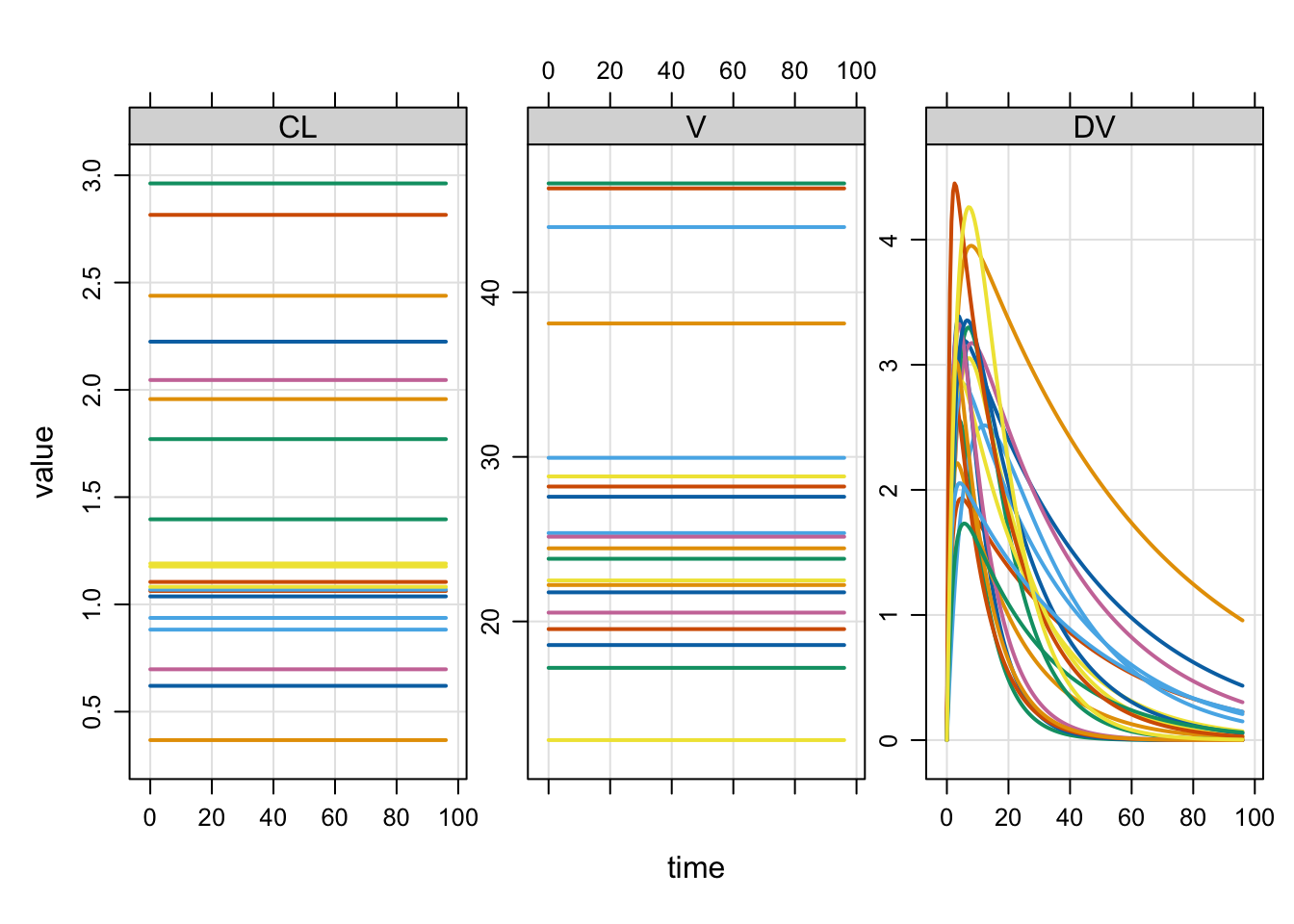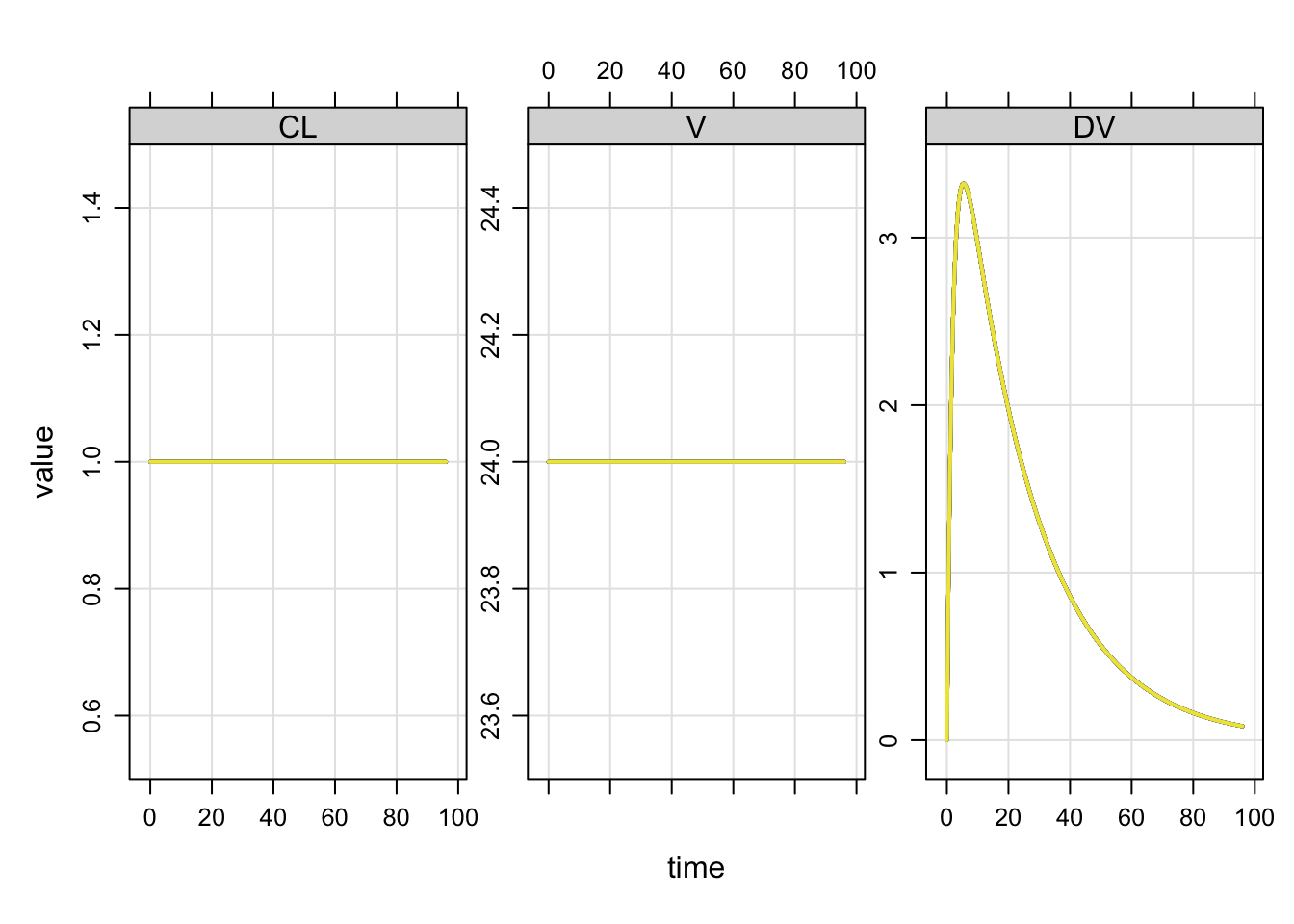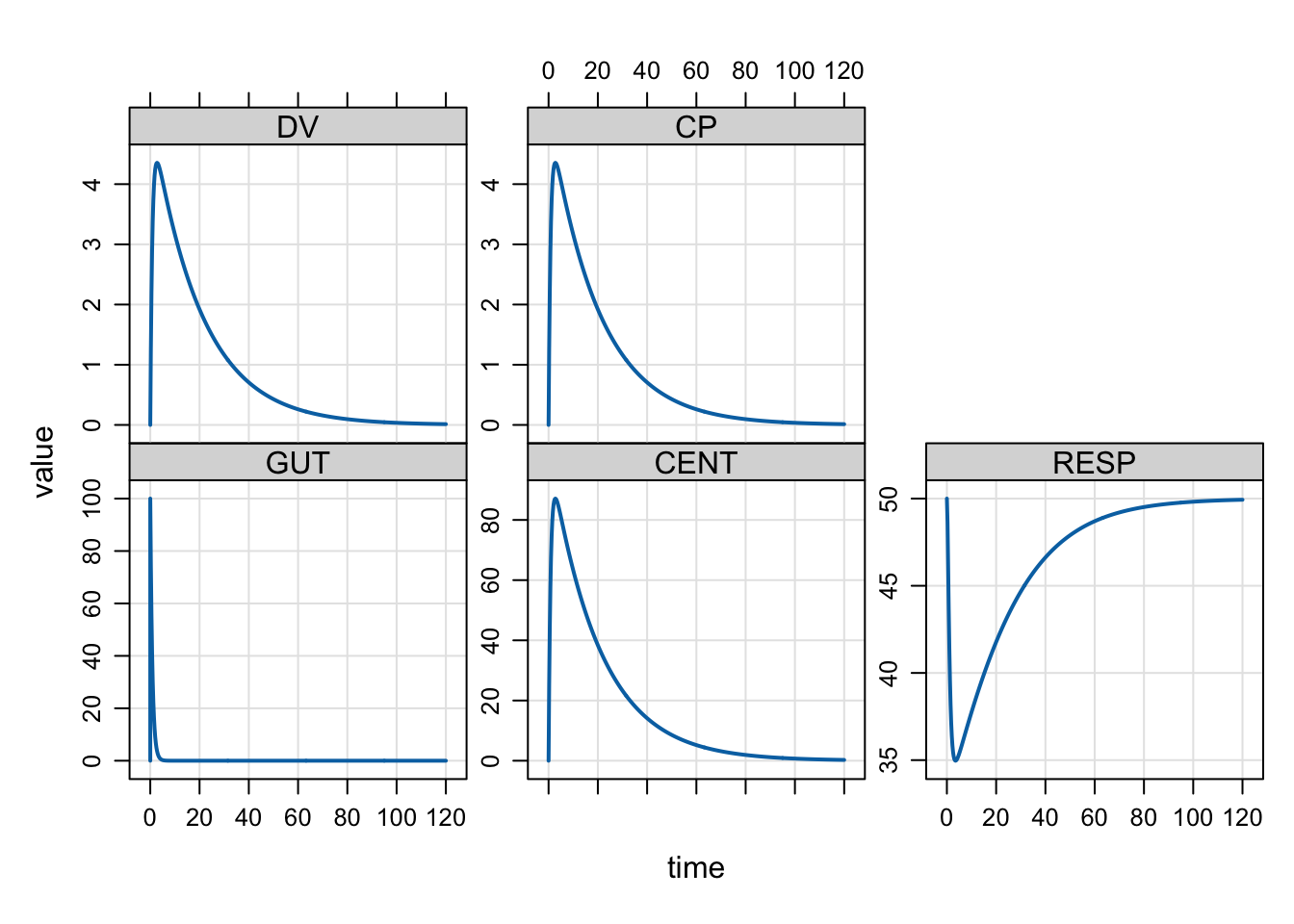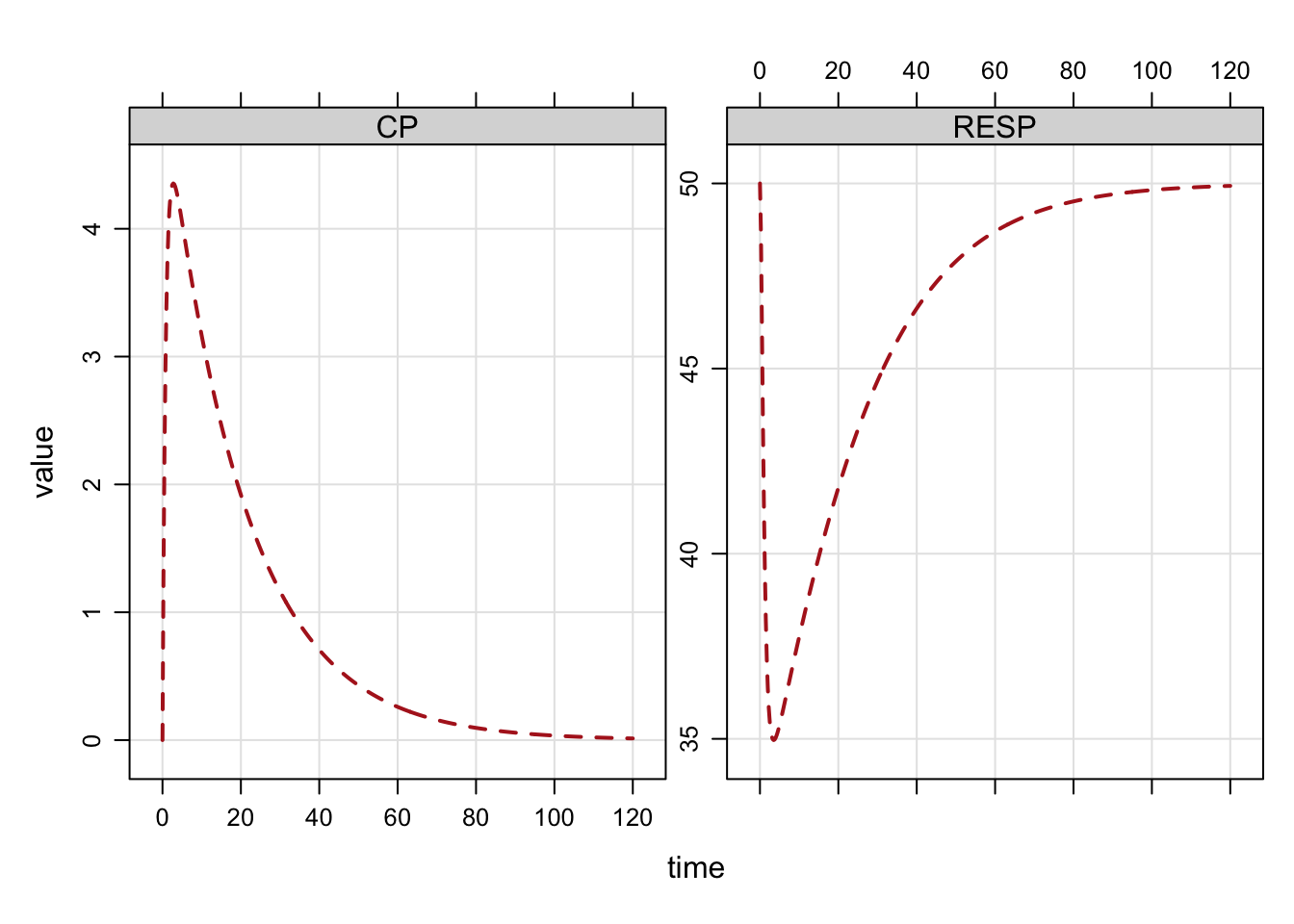# Quick hit demos

These are all miscellaneous features that may be difficult to find in other documentation.

``````library(mrgsolve)
library(dplyr)``````

# `\$` operator for model object gets the parameter value

If our model parameters are

``````mod <- mrgsolve:::house()

param(mod)``````
``````
Model parameters (N=14):
name value . name  value
CL   1     | SEX   0
D1   2     | SEXCL 0.7
F1   1     | SEXVC 0.85
IC50 10    | VC    20
KA   1.2   | WT    70
KIN  100   | WTCL  0.75
KOUT 2     | WTVC  1    ``````

we can pick a parameter value with

``mod\$CL``
`` 1``
``mod\$WT``
`` 70``

Or slice off multiple parameters

``````i_want <- c("CL", "WT")
mod[i_want]``````
``````\$CL
 1

\$WT
 70``````

# Model names

For programming with a model object, we can extract the names

``names(mod)``
``````\$param
 "CL"    "VC"    "KA"    "F1"    "D1"    "WT"    "SEX"   "WTCL"  "WTVC"
 "SEXCL" "SEXVC" "KIN"   "KOUT"  "IC50"

\$init
 "GUT"  "CENT" "RESP"

\$capture
 "DV" "CP"

\$omega
 "..."

\$sigma
 "..."

\$omega_labels
\$omega_labels[]
 "ECL"   "EVC"   "EKA"   "EKOUT"

\$sigma_labels
\$sigma_labels[]
 "EXPO"``````

or get all of the model elements in a list (output not shown)

``my_model <- as.list(mod)``

# Zero all random effect variances on the fly

If your model has random effects, you can easily and temporarily zero them out.

``mod <- modlib("popex") %>% update(end=96) %>% Req(DV,CL,V)``
``Building popex ... done.``
``omat(mod)``
``````\$...
[,1] [,2] [,3]
ECL:   0.3  0.0  0.0
EV:    0.0  0.1  0.0
EKA:   0.0  0.0  0.5``````

It is easy to simulate either with or without the random effects in the simulation: this change can be made on the fly.

Use `zero_re` to make all random effect variances zero

``mod %>% zero_re %>% omat``
``````\$...
[,1] [,2] [,3]
ECL:     0    0    0
EV:      0    0    0
EKA:     0    0    0``````

By default, both `OMEGA` and `SIGMA` are zeroed. Check the arguments for `zero_re` to see how to selectively zero `OMEGA` or `SIGMA`.

Compare the population output

``mod %>% ev(amt=100) %>% mrgsim(nid=20) %>% plot``with

``mod %>% ev(amt=100) %>% zero_re %>% mrgsim(nid=20) %>% plot``# Plot formulae

We commonly plot simulated output with a special plot method. By default, you get all compartments and output variables in the plot.

``````mod <- mrgsolve:::house() %>% ev(amt=100)
mod %>% mrgsim %>% plot``````The plot can be customized with a formula selecting variables to plot. Other arguments

to `lattice::xyplot` can be passed as well.

``mod %>% mrgsim %>% plot(CP+RESP ~ time, lty=2, col="firebrick")``# Get a data frame of simulated data

By default mrgsolve returns an object of simulated data (and other stuff)

``````out <- mrgsim(mod)

class(out)``````
`````` "mrgsims"
attr(,"package")
 "mrgsolve"``````

But you can get a data frame with

``````out <- mrgsim_df(mod)

class(out)``````
`` "data.frame"``

or

``````out <- mrgsim(mod,output="df")

out <- mrgsim(mod) %>% as_tibble()``````